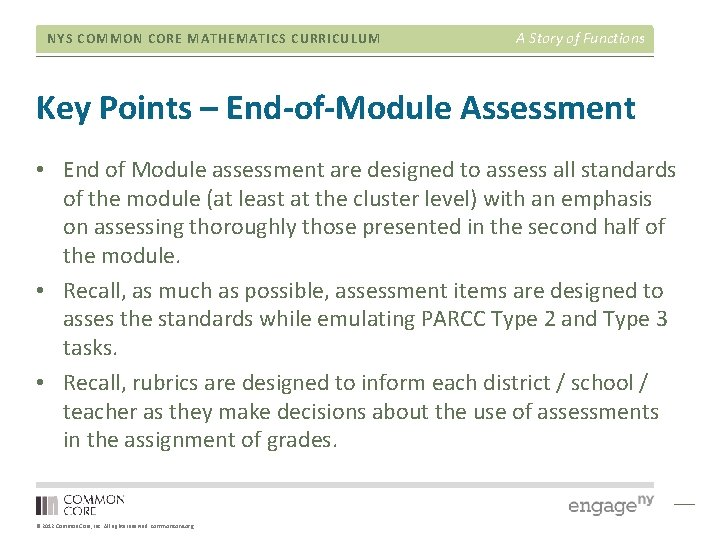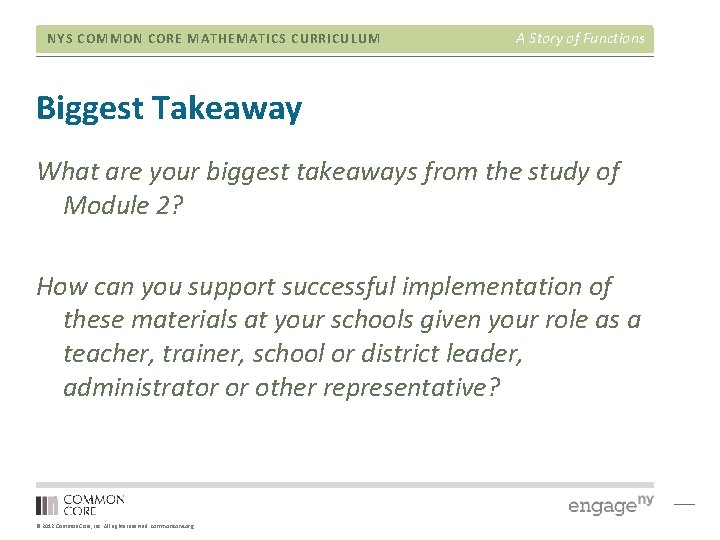# NYS COMMON CORE MATHEMATICS CURRICULUM A Story of

• Slides: 51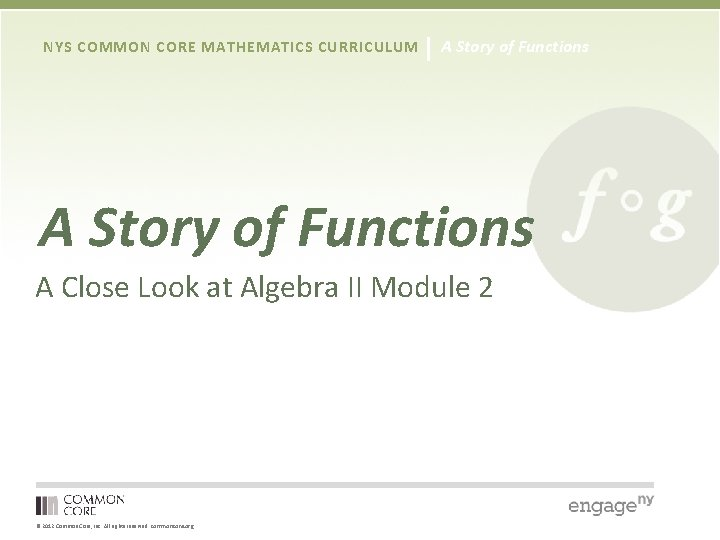NYS COMMON CORE MATHEMATICS CURRICULUM A Story of Functions A Close Look at Algebra II Module 2 © 2012 Common Core, Inc. All rights reserved. commoncore. org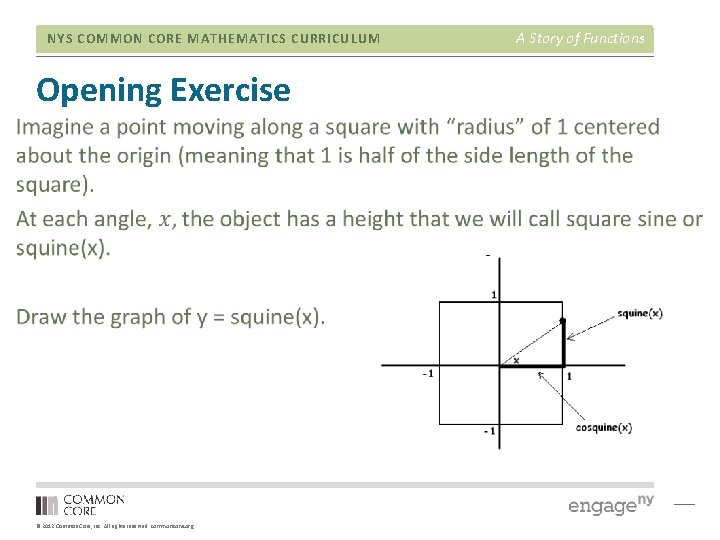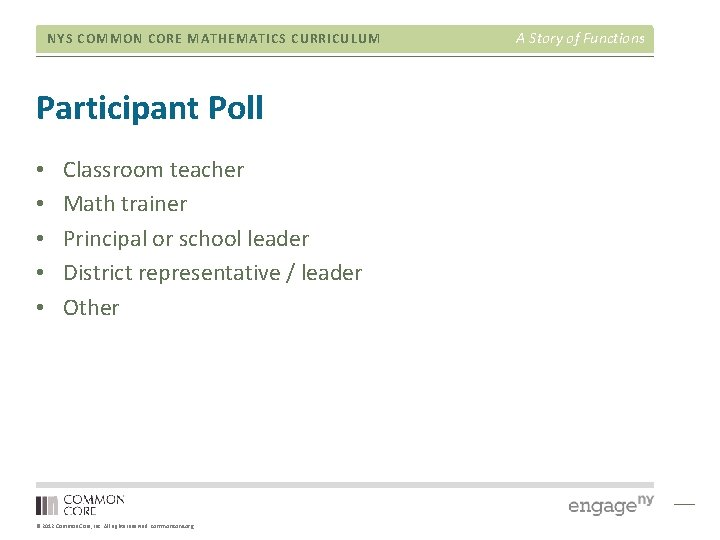NYS COMMON CORE MATHEMATICS CURRICULUM Participant Poll • • • Classroom teacher Math trainer Principal or school leader District representative / leader Other © 2012 Common Core, Inc. All rights reserved. commoncore. org A Story of Functions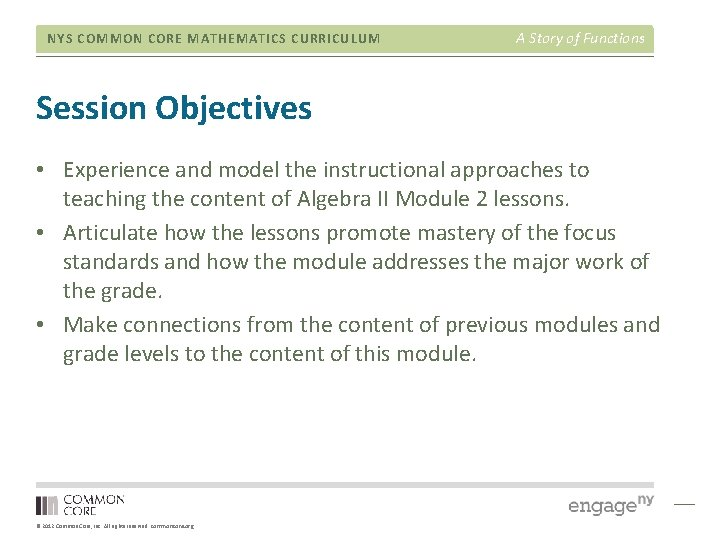NYS COMMON CORE MATHEMATICS CURRICULUM A Story of Functions Session Objectives • Experience and model the instructional approaches to teaching the content of Algebra II Module 2 lessons. • Articulate how the lessons promote mastery of the focus standards and how the module addresses the major work of the grade. • Make connections from the content of previous modules and grade levels to the content of this module. © 2012 Common Core, Inc. All rights reserved. commoncore. orgNYS COMMON CORE MATHEMATICS CURRICULUM Agenda • • • Overview of Module 2 Topic A Mid-Module Assessment Topic B End of Module Assessment © 2012 Common Core, Inc. All rights reserved. commoncore. org A Story of FunctionsNYS COMMON CORE MATHEMATICS CURRICULUM A Story of Functions Flow of Module 2 • 17 lessons • 7 exploration • 6 socratic • 4 problem set • 21 instructional days • Topic A: The Story of Trigonometry and Its Context • Topic B: Understanding Trigonometric Functions and Putting Them to Use © 2012 Common Core, Inc. All rights reserved. commoncore. orgNYS COMMON CORE MATHEMATICS CURRICULUM A Story of Functions Mathematical Themes of Module 2 • Emphasis on exploratory lessons rather than problem set lessons • Developing the sine and cosine functions through exploration of the horizontal and vertical displacement of a car on a rotating Ferris wheel • Defining sine and cosine as functions of real numbers • Graphing the sine and cosine functions and transformations of these functions • Discovering and proving trigonometric identities © 2012 Common Core, Inc. All rights reserved. commoncore. org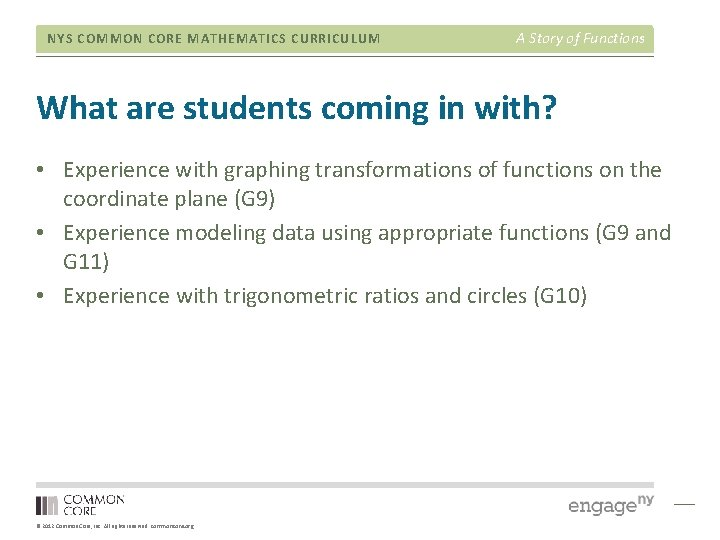NYS COMMON CORE MATHEMATICS CURRICULUM A Story of Functions What are students coming in with? • Experience with graphing transformations of functions on the coordinate plane (G 9) • Experience modeling data using appropriate functions (G 9 and G 11) • Experience with trigonometric ratios and circles (G 10) © 2012 Common Core, Inc. All rights reserved. commoncore. org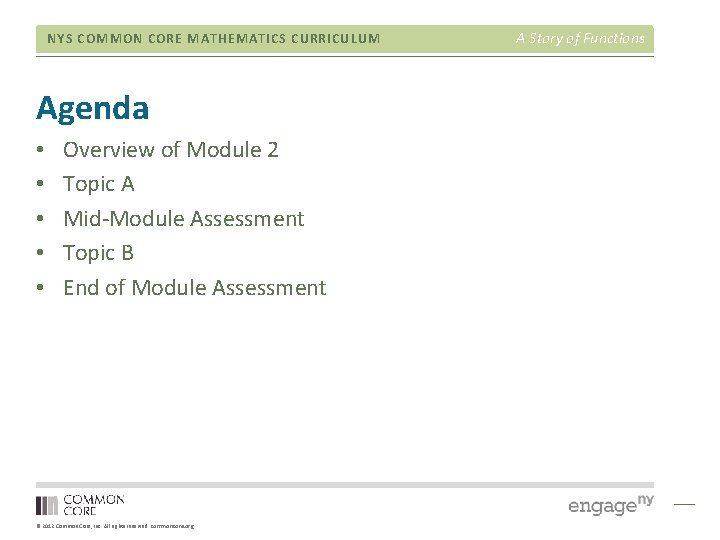NYS COMMON CORE MATHEMATICS CURRICULUM Agenda • • • Overview of Module 2 Topic A Mid-Module Assessment Topic B End of Module Assessment © 2012 Common Core, Inc. All rights reserved. commoncore. org A Story of Functions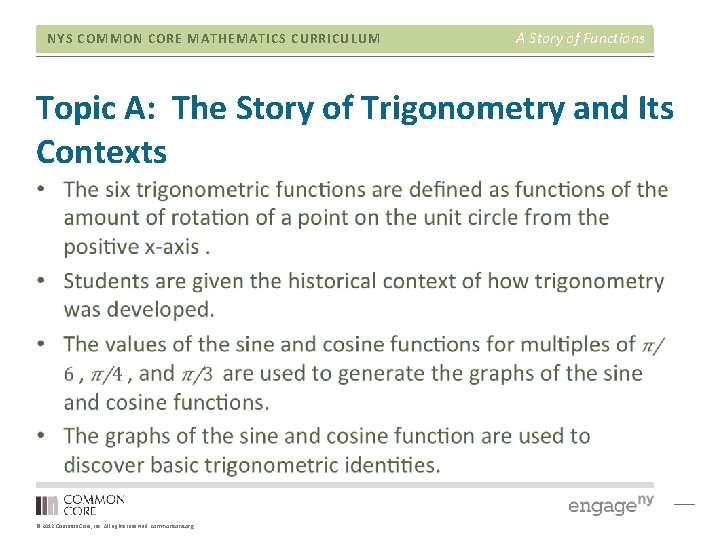NYS COMMON CORE MATHEMATICS CURRICULUM A Story of Functions Topic A: The Story of Trigonometry and Its Contexts © 2012 Common Core, Inc. All rights reserved. commoncore. org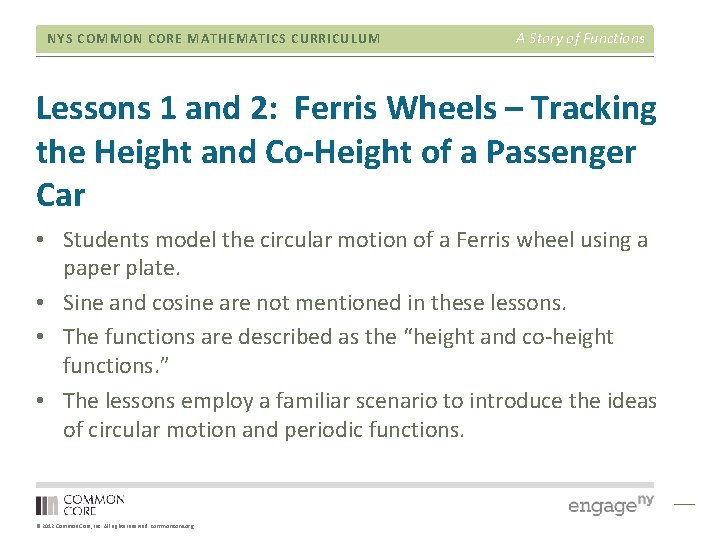NYS COMMON CORE MATHEMATICS CURRICULUM A Story of Functions Lessons 1 and 2: Ferris Wheels – Tracking the Height and Co-Height of a Passenger Car • Students model the circular motion of a Ferris wheel using a paper plate. • Sine and cosine are not mentioned in these lessons. • The functions are described as the “height and co-height functions. ” • The lessons employ a familiar scenario to introduce the ideas of circular motion and periodic functions. © 2012 Common Core, Inc. All rights reserved. commoncore. org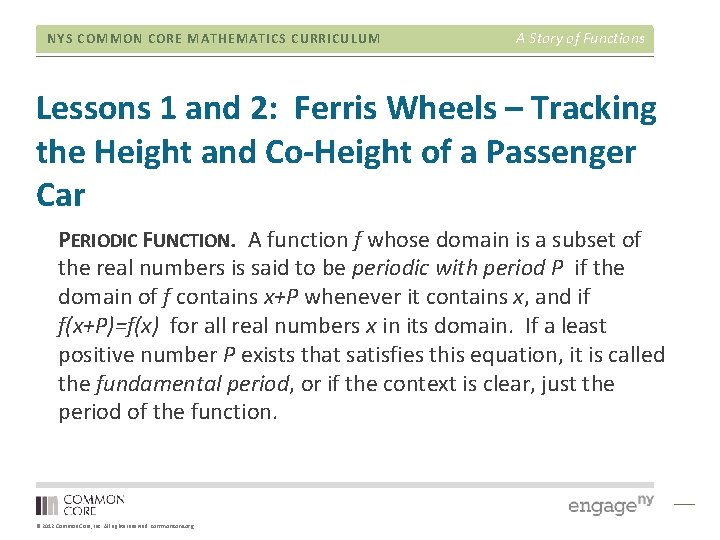NYS COMMON CORE MATHEMATICS CURRICULUM A Story of Functions Lessons 1 and 2: Ferris Wheels – Tracking the Height and Co-Height of a Passenger Car PERIODIC FUNCTION. A function f whose domain is a subset of the real numbers is said to be periodic with period P if the domain of f contains x+P whenever it contains x, and if f(x+P)=f(x) for all real numbers x in its domain. If a least positive number P exists that satisfies this equation, it is called the fundamental period, or if the context is clear, just the period of the function. © 2012 Common Core, Inc. All rights reserved. commoncore. orgNYS COMMON CORE MATHEMATICS CURRICULUM A Story of Functions Lessons 3: The Motion of the Moon, Sun, and Stars—Motivating Mathematics © 2012 Common Core, Inc. All rights reserved. commoncore. orgNYS COMMON CORE MATHEMATICS CURRICULUM A Story of Functions Lessons 4: From Circle-ometry to Trigonometry • • Work example 1 and 2. Work problem set 2 – 5. Lots of information to process in this lesson. Consider using the scaffold suggestion of creating a graphic organizer. © 2012 Common Core, Inc. All rights reserved. commoncore. orgNYS COMMON CORE MATHEMATICS CURRICULUM A Story of Functions Lessons 4: From Circle-ometry to Trigonometry © 2012 Common Core, Inc. All rights reserved. commoncore. org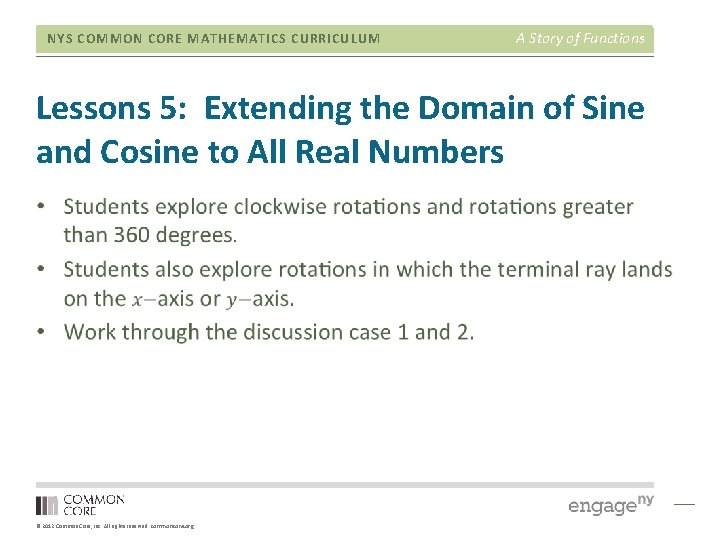NYS COMMON CORE MATHEMATICS CURRICULUM A Story of Functions Lessons 5: Extending the Domain of Sine and Cosine to All Real Numbers © 2012 Common Core, Inc. All rights reserved. commoncore. orgNYS COMMON CORE MATHEMATICS CURRICULUM A Story of Functions Lesson 6: Why Call it Tangent? • Work the opening exercise. • You may need to review some terminology from geometry. © 2012 Common Core, Inc. All rights reserved. commoncore. orgNYS COMMON CORE MATHEMATICS CURRICULUM Lesson 6: Why Call it Tangent? • Students interpret the tangent function several ways: © 2012 Common Core, Inc. All rights reserved. commoncore. org A Story of Functions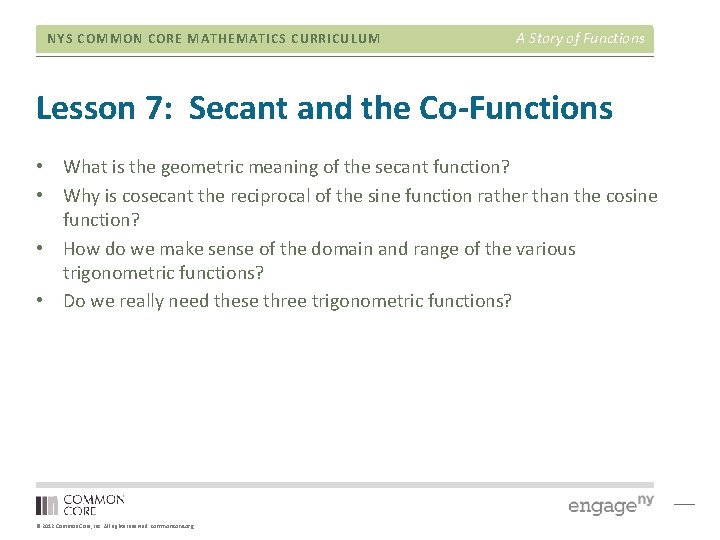NYS COMMON CORE MATHEMATICS CURRICULUM A Story of Functions Lesson 7: Secant and the Co-Functions • What is the geometric meaning of the secant function? • Why is cosecant the reciprocal of the sine function rather than the cosine function? • How do we make sense of the domain and range of the various trigonometric functions? • Do we really need these three trigonometric functions? © 2012 Common Core, Inc. All rights reserved. commoncore. org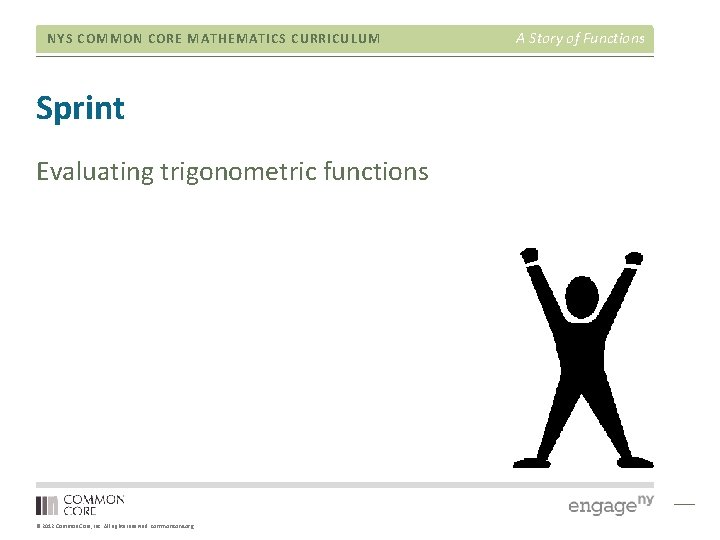NYS COMMON CORE MATHEMATICS CURRICULUM Sprint Evaluating trigonometric functions © 2012 Common Core, Inc. All rights reserved. commoncore. org A Story of FunctionsNYS COMMON CORE MATHEMATICS CURRICULUM A Story of Functions Lesson 8: Graphing the Sine and Cosine Functions • Students construct the graphs of f and g first by using the vertical and horizontal components of points on the unit circle and then by using the known values of sine and cosine. • The emphasis should be on the key features of each graph. • • Relative maxima and minima Intervals of increasing and decreasing Intercepts End behavior Symmetry Amplitude Period © 2012 Common Core, Inc. All rights reserved. commoncore. org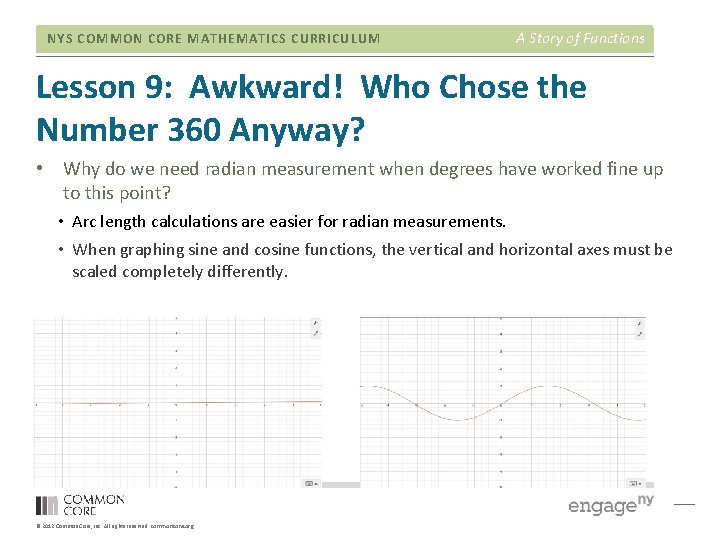NYS COMMON CORE MATHEMATICS CURRICULUM A Story of Functions Lesson 9: Awkward! Who Chose the Number 360 Anyway? • Why do we need radian measurement when degrees have worked fine up to this point? • Arc length calculations are easier for radian measurements. • When graphing sine and cosine functions, the vertical and horizontal axes must be scaled completely differently. © 2012 Common Core, Inc. All rights reserved. commoncore. org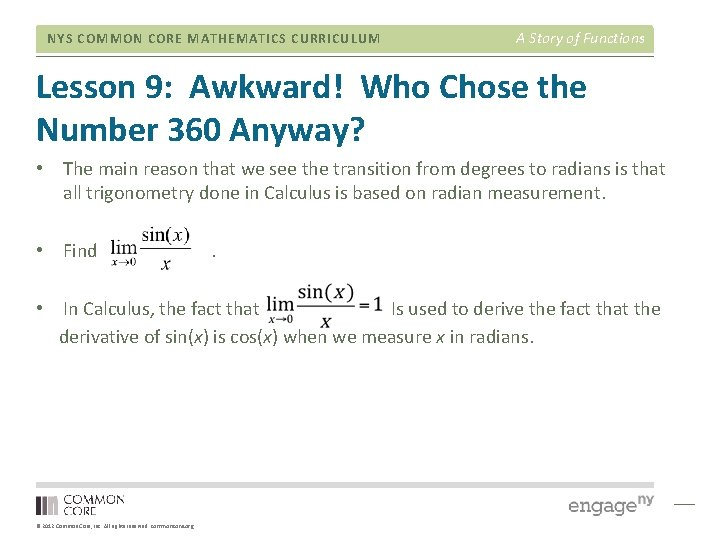NYS COMMON CORE MATHEMATICS CURRICULUM A Story of Functions Lesson 9: Awkward! Who Chose the Number 360 Anyway? • The main reason that we see the transition from degrees to radians is that all trigonometry done in Calculus is based on radian measurement. • Find . • In Calculus, the fact that Is used to derive the fact that the derivative of sin(x) is cos(x) when we measure x in radians. © 2012 Common Core, Inc. All rights reserved. commoncore. org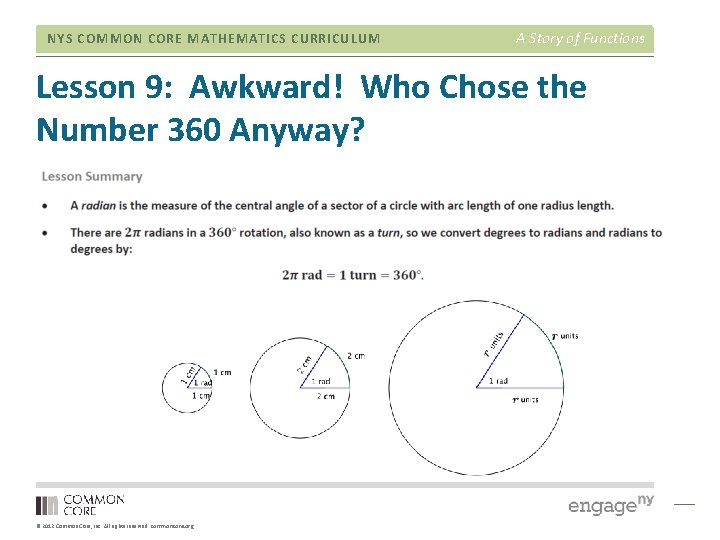NYS COMMON CORE MATHEMATICS CURRICULUM A Story of Functions Lesson 9: Awkward! Who Chose the Number 360 Anyway? © 2012 Common Core, Inc. All rights reserved. commoncore. orgNYS COMMON CORE MATHEMATICS CURRICULUM A Story of Functions Lesson 9: Awkward! Who Chose the Number 360 Anyway? © 2012 Common Core, Inc. All rights reserved. commoncore. orgNYS COMMON CORE MATHEMATICS CURRICULUM A Story of Functions Lesson 10: Basic Trigonometric Identities from Graphs • Students use the graphs of the sine and cosine functions to note properties that lead to trigonometric identities. • Periodicity • Symmetry (even/odd functions) • Horizontal translations • Note that an identity is a statement that two functions are equal on a common domain. • The statement “sin(x+2π)=sin(x)” itself is not an identity. • The correct statement is “sin(x+2π)=sin(x) for all real numbers x. ” © 2012 Common Core, Inc. All rights reserved. commoncore. org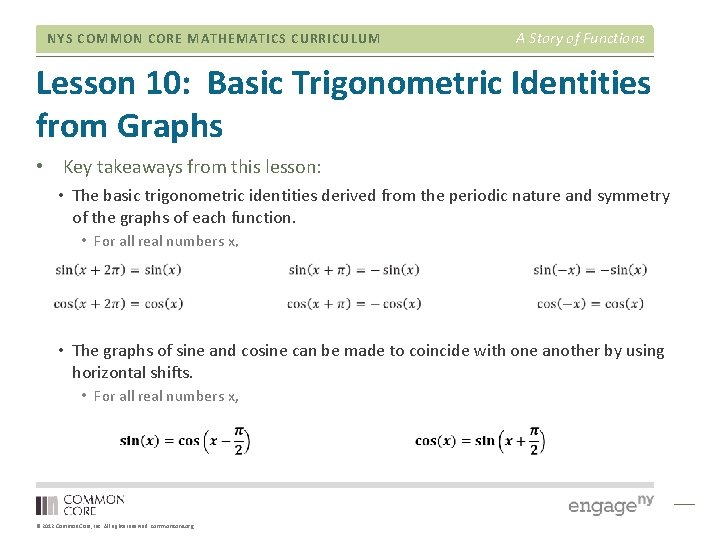NYS COMMON CORE MATHEMATICS CURRICULUM A Story of Functions Lesson 10: Basic Trigonometric Identities from Graphs • Key takeaways from this lesson: • The basic trigonometric identities derived from the periodic nature and symmetry of the graphs of each function. • For all real numbers x, • The graphs of sine and cosine can be made to coincide with one another by using horizontal shifts. • For all real numbers x, © 2012 Common Core, Inc. All rights reserved. commoncore. org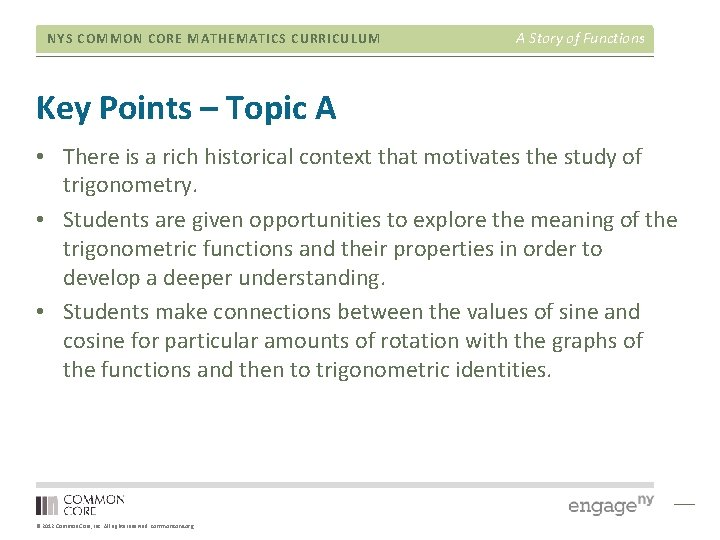NYS COMMON CORE MATHEMATICS CURRICULUM A Story of Functions Key Points – Topic A • There is a rich historical context that motivates the study of trigonometry. • Students are given opportunities to explore the meaning of the trigonometric functions and their properties in order to develop a deeper understanding. • Students make connections between the values of sine and cosine for particular amounts of rotation with the graphs of the functions and then to trigonometric identities. © 2012 Common Core, Inc. All rights reserved. commoncore. orgNYS COMMON CORE MATHEMATICS CURRICULUM Agenda • • • Overview of Module 2 Topic A Mid-Module Assessment Topic B End of Module Assessment © 2012 Common Core, Inc. All rights reserved. commoncore. org A Story of Functions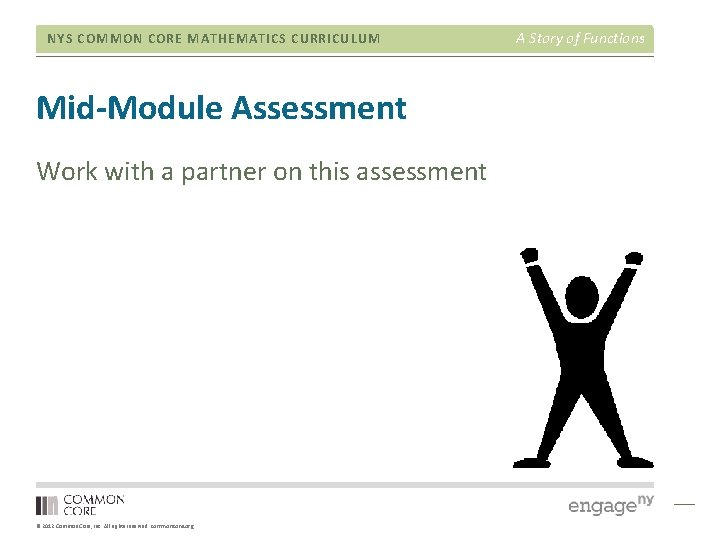NYS COMMON CORE MATHEMATICS CURRICULUM Mid-Module Assessment Work with a partner on this assessment © 2012 Common Core, Inc. All rights reserved. commoncore. org A Story of Functions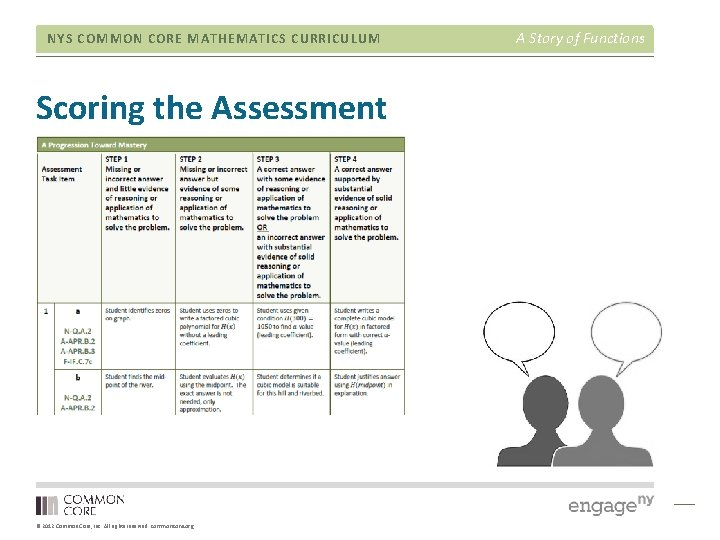NYS COMMON CORE MATHEMATICS CURRICULUM Agenda • • • Overview of Module 2 Topic A Mid-Module Assessment Topic B End of Module Assessment © 2012 Common Core, Inc. All rights reserved. commoncore. org A Story of Functions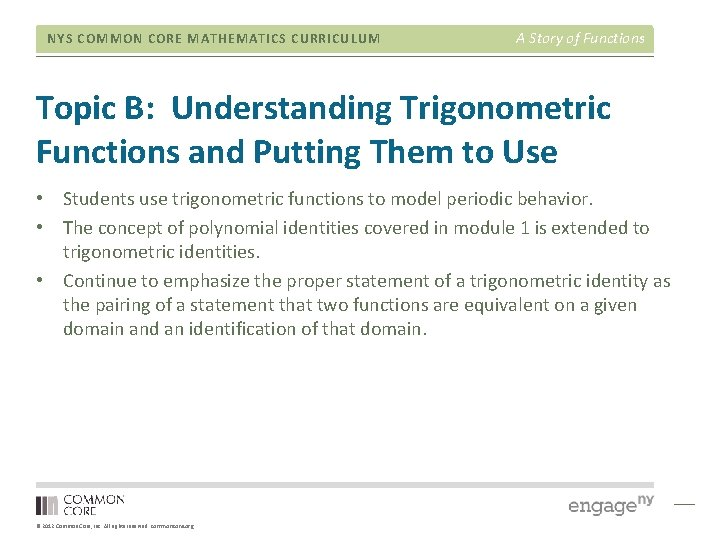NYS COMMON CORE MATHEMATICS CURRICULUM A Story of Functions Topic B: Understanding Trigonometric Functions and Putting Them to Use • Students use trigonometric functions to model periodic behavior. • The concept of polynomial identities covered in module 1 is extended to trigonometric identities. • Continue to emphasize the proper statement of a trigonometric identity as the pairing of a statement that two functions are equivalent on a given domain and an identification of that domain. © 2012 Common Core, Inc. All rights reserved. commoncore. org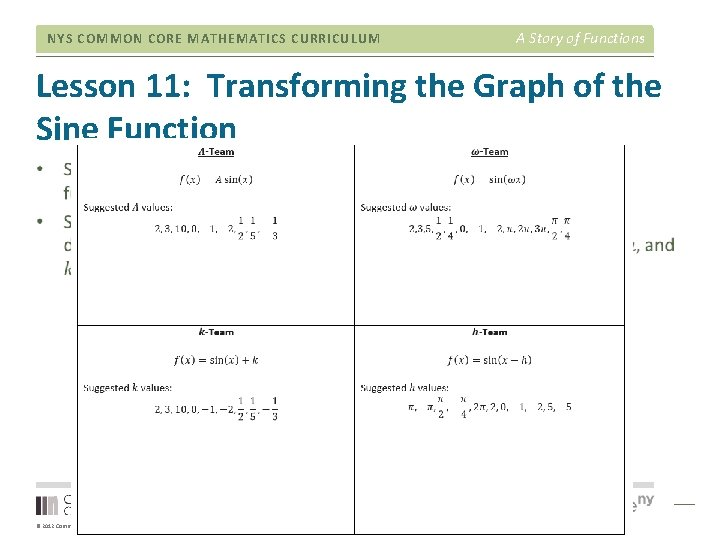NYS COMMON CORE MATHEMATICS CURRICULUM A Story of Functions Lesson 11: Transforming the Graph of the Sine Function © 2012 Common Core, Inc. All rights reserved. commoncore. orgNYS COMMON CORE MATHEMATICS CURRICULUM A Story of Functions Lesson 11: Transforming the Graph of the Sine Function © 2012 Common Core, Inc. All rights reserved. commoncore. org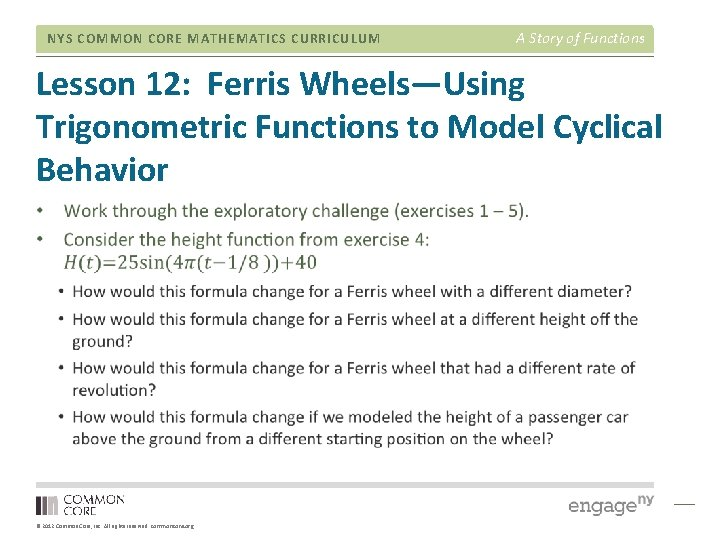NYS COMMON CORE MATHEMATICS CURRICULUM A Story of Functions Lesson 12: Ferris Wheels—Using Trigonometric Functions to Model Cyclical Behavior © 2012 Common Core, Inc. All rights reserved. commoncore. org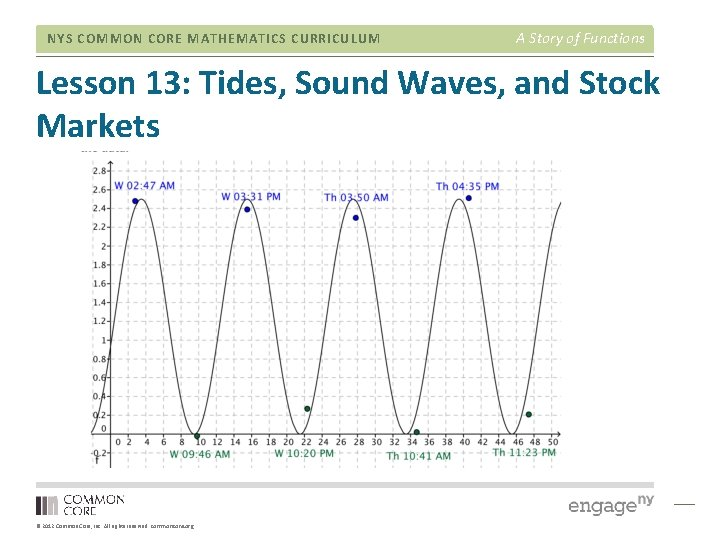NYS COMMON CORE MATHEMATICS CURRICULUM A Story of Functions Lesson 13: Tides, Sound Waves, and Stock Markets © 2012 Common Core, Inc. All rights reserved. commoncore. org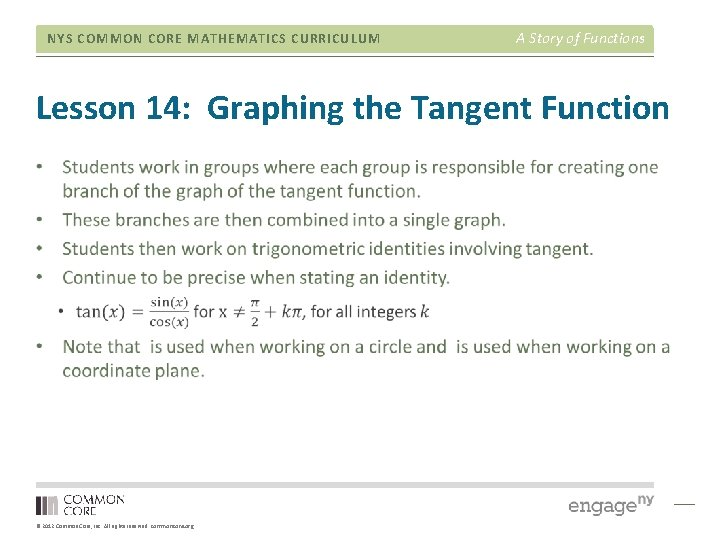NYS COMMON CORE MATHEMATICS CURRICULUM A Story of Functions Lesson 14: Graphing the Tangent Function © 2012 Common Core, Inc. All rights reserved. commoncore. org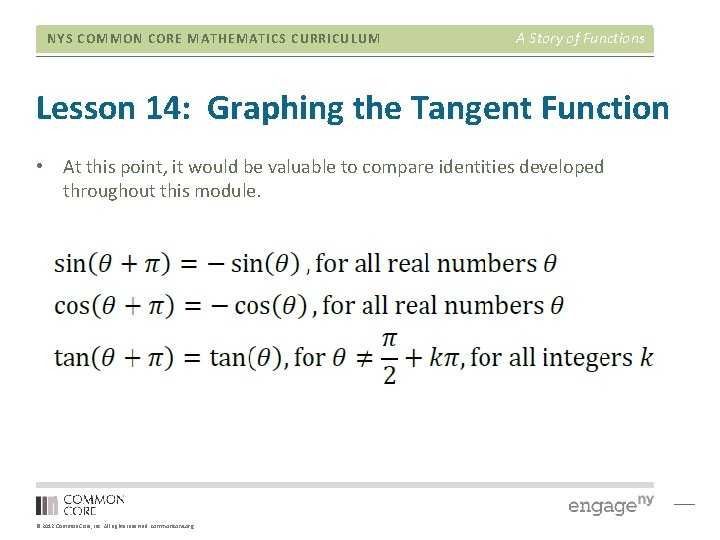NYS COMMON CORE MATHEMATICS CURRICULUM A Story of Functions Lesson 14: Graphing the Tangent Function • At this point, it would be valuable to compare identities developed throughout this module. © 2012 Common Core, Inc. All rights reserved. commoncore. org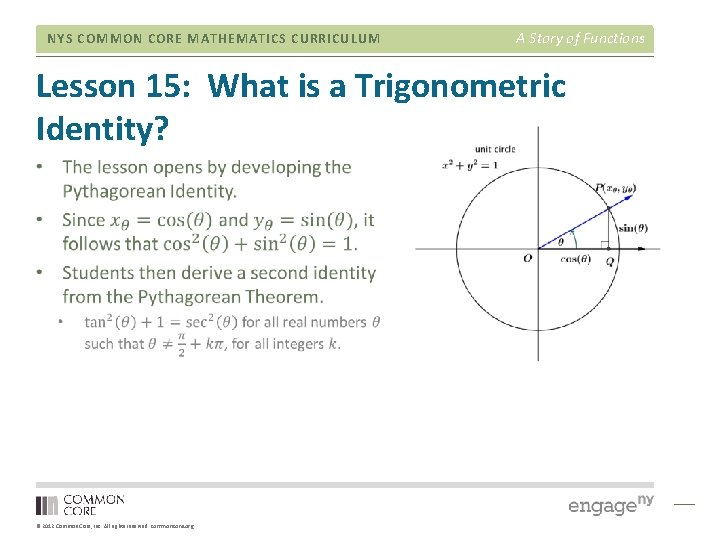NYS COMMON CORE MATHEMATICS CURRICULUM A Story of Functions Lesson 15: What is a Trigonometric Identity? © 2012 Common Core, Inc. All rights reserved. commoncore. org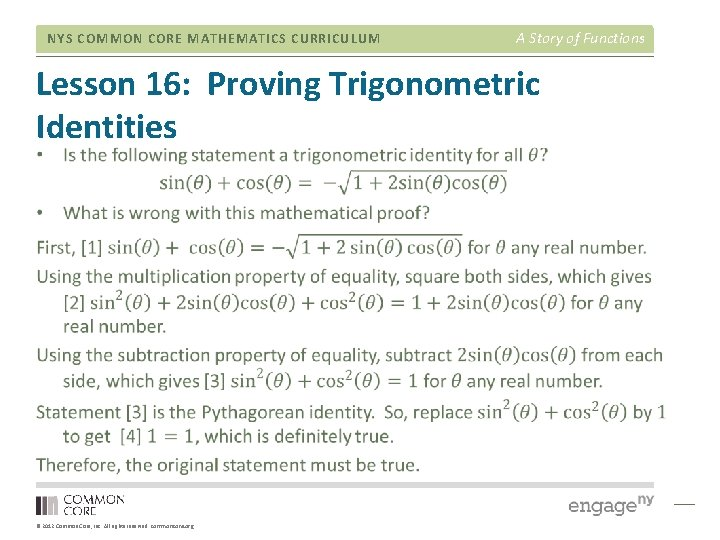NYS COMMON CORE MATHEMATICS CURRICULUM A Story of Functions Lesson 16: Proving Trigonometric Identities © 2012 Common Core, Inc. All rights reserved. commoncore. org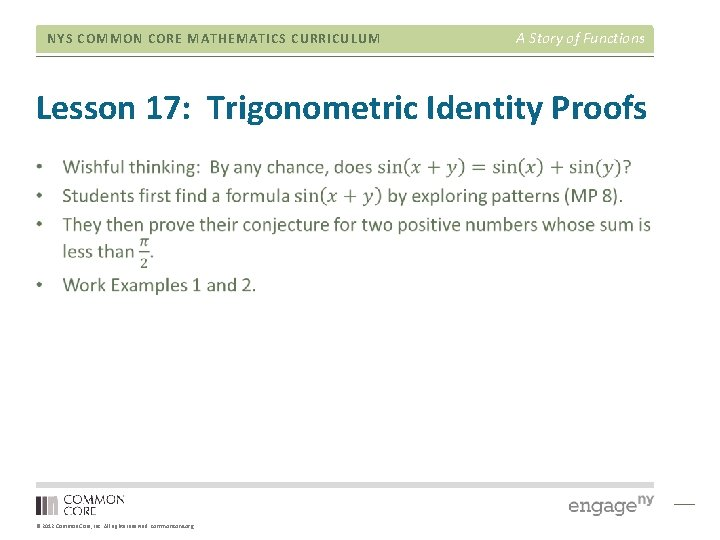NYS COMMON CORE MATHEMATICS CURRICULUM A Story of Functions Lesson 17: Trigonometric Identity Proofs © 2012 Common Core, Inc. All rights reserved. commoncore. org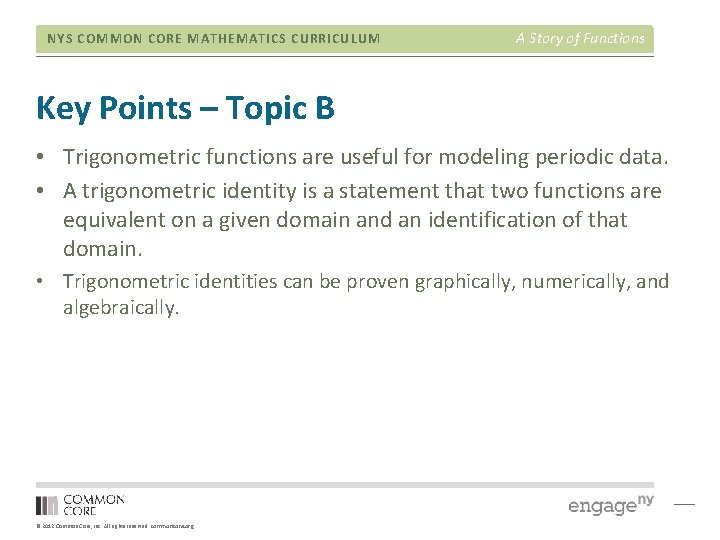NYS COMMON CORE MATHEMATICS CURRICULUM A Story of Functions Key Points – Topic B • Trigonometric functions are useful for modeling periodic data. • A trigonometric identity is a statement that two functions are equivalent on a given domain and an identification of that domain. • Trigonometric identities can be proven graphically, numerically, and algebraically. © 2012 Common Core, Inc. All rights reserved. commoncore. orgNYS COMMON CORE MATHEMATICS CURRICULUM Key Points – Module 2 Lessons © 2012 Common Core, Inc. All rights reserved. commoncore. org A Story of FunctionsNYS COMMON CORE MATHEMATICS CURRICULUM Agenda • • • Overview of Module 2 Topic A Mid-Module Assessment Topic B End of Module Assessment © 2012 Common Core, Inc. All rights reserved. commoncore. org A Story of Functions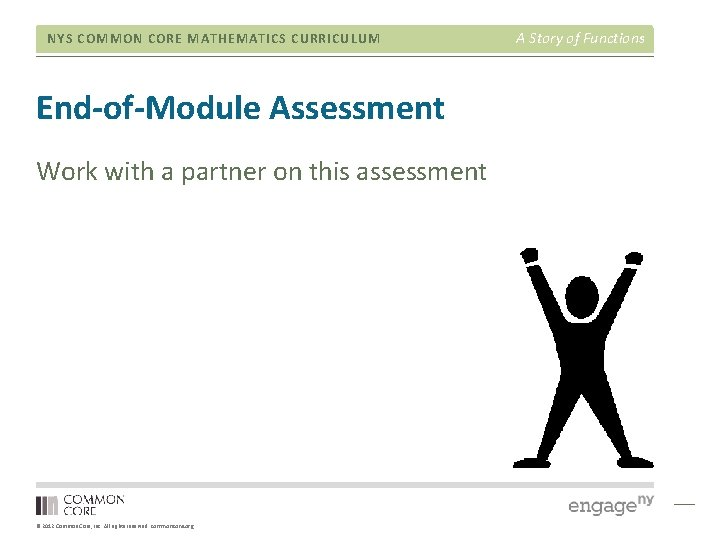NYS COMMON CORE MATHEMATICS CURRICULUM End-of-Module Assessment Work with a partner on this assessment © 2012 Common Core, Inc. All rights reserved. commoncore. org A Story of Functions